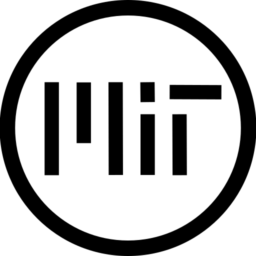# Ray-box setup

Ray setup and useful intersections with a bounding box in model space, ideal for ray marching. You may build on this code to cast rays.

I couldn’t find any examples that support orthographic and VR mode out of the gate, so here you go. It can be an annoying roadblock when you just want to implement your ray based algorithm and get results.

The shader is designed for use in Godot 4.

Setup

1. Add a new MeshInstance3D node into the scene
2. Assign it a BoxMesh
3. Set the width, height and depth to 2
4. Set `flip_faces`=`true`

I hope this helps someone. Please let me know if you find a better approach!

P.S.

The ray-box intersector is lifted from https://iquilezles.org/articles/intersectors/

I did derive my own, but the formula ended up being identical to iq anyway…

``````shader_type spatial;

// axis aligned box centered at the origin, with size boxSize
vec2 boxIntersection( in vec3 ro, in vec3 rd, vec3 boxSize, out vec3 outNormal )
{
vec3 m = 1.0/rd; // can precompute if traversing a set of aligned boxes
vec3 n = m*ro;   // can precompute if traversing a set of aligned boxes
vec3 k = abs(m)*boxSize;
vec3 t1 = -n - k;
vec3 t2 = -n + k;
float tN = max( max( t1.x, t1.y ), t1.z );
float tF = min( min( t2.x, t2.y ), t2.z );
if( tN>tF || tF<0.0) return vec2(-1.0); // no intersection
outNormal = (tN>0.0) ? step(vec3(tN),t1) : // ro ouside the box
step(t2,vec3(tF));  // ro inside the box
outNormal *= -sign(rd);
return vec2( tN, tF );
}

void fragment() {
// construct the ray in view space
vec3 rd = -VIEW;
vec3 ro = VERTEX - rd * VERTEX.z/rd.z;

// transform ray to model space
mat4 inv_modelview = inverse(VIEW_MATRIX * MODEL_MATRIX);
ro = (inv_modelview * vec4(ro,1)).xyz;
rd = normalize( mat3(inv_modelview) * rd );

// intersect with the box
vec3 n;
vec2 result = boxIntersection(ro, rd, vec3(1), n);

// get the first point along the ray that is inside of the box
float t = max(result.x, 0.0);
vec3 p = ro + rd * t;

// <-- INSERT RAY BASED ALGORITHM

// discard any rays that made it all the way through the box

// debugging
ALBEDO = vec3(p);
}``````
###### Tags
box, godot 4, ray, raymaching, templateThe shader code and all code snippets in this post are under MIT license and can be used freely. Images and videos, and assets depicted in those, do not fall under this license. For more info, see our License terms.

## More from celyk

### Focus rectangle / box

Subscribe
Notify of1 Comment
Inline Feedbacks